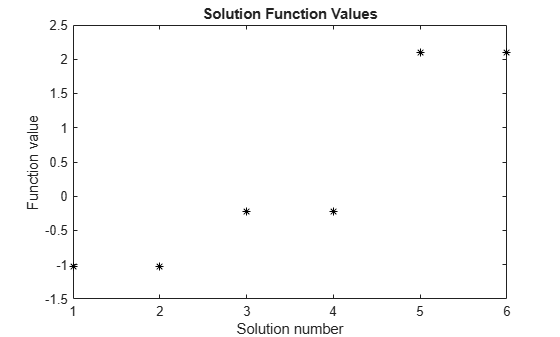# GlobalOptimSolution

Optimization solution

## Description

A `GlobalOptimSolution` object contains information on a local minimum, including location, objective function value, and start point or points that lead to the minimum.

`GlobalSearch` and `MultiStart` generate a vector of `GlobalOptimSolution` objects. The vector is ordered by objective function value, from lowest (best) to highest (worst). `GlobalSearch` and `MultiStart` combine solutions that coincide with previously found solutions to within tolerances. For `GlobalSearch` details, see Update Solution Set in When fmincon Runs. For `MultiStart` details, see Create GlobalOptimSolution Object.

## Creation

When you execute `run` and request the solutions output, `GlobalSearch` and `MultiStart` create `GlobalOptimSolution` objects as output.

## Properties

expand all

Exit condition of the local solver, returned as an integer. Generally, a positive `Exitflag` corresponds to a local optimum, and a zero or negative `Exitflag` corresponds to an unsuccessful search for a local minimum.

For the exact meaning of each `Exitflag`, see the `exitflag` description in the appropriate local solver function reference page:

Data Types: `double`

Objective function value, returned as a real scalar. For the `lsqnonlin` and `lsqcurvefit` solvers, `Fval` is the sum of squares of the residual.

Data Types: `double`

Output structure returned by the local solver. For details, see the `output` description in the appropriate local solver function reference page:

Data Types: `struct`

Local solution, returned as an array with the same dimensions as `problem.x0`.

Data Types: `double`

Start points that lead to current solution, returned as a cell array. Control the distance between points considered as distinct by setting the `FunctionTolerance` and `XTolerance` properties of the global solver.

Data Types: `cell`

## Examples

collapse all

Use `MultiStart` to create a vector of `GlobalOptimSolution` objects for a problem with multiple local minima.

```rng default % For reproducibility ms = MultiStart; sixmin = @(x)(4*x(1)^2 - 2.1*x(1)^4 + x(1)^6/3 ... + x(1)*x(2) - 4*x(2)^2 + 4*x(2)^4); problem = createOptimProblem('fmincon','x0',[-1,2],... 'objective',sixmin,'lb',[-3,-3],'ub',[3,3]); [xmin,fmin,flag,outpt,allmins] = run(ms,problem,30);```
```MultiStart completed the runs from all start points. All 30 local solver runs converged with a positive local solver exit flag. ```

`allmins` is a vector of `GlobalOptimSolution` objects.

`disp(allmins)`
``` 1x6 GlobalOptimSolution array with properties: X Fval Exitflag Output X0 ```

Plot the objective function values at the returned solutions.

```plot(arrayfun(@(x)x.Fval,allmins),'k*') xlabel('Solution number') ylabel('Function value') title('Solution Function Values')```To examine the initial points that lead to the various solutions, see Visualize the Basins of Attraction.

### Topics

Introduced in R2010a

## Support평가판 신청